## 7.13Thermal boundary layers

In a turbulent boundary layer, the distribution of temperature is similar to velocity, with the viscous and intertial sub-layers, separated by a buﬀer layer, as discussed in Sec. 7.4 .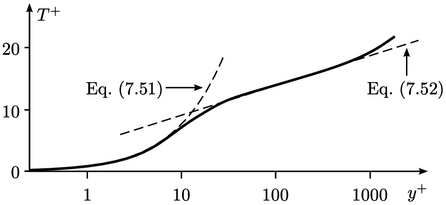By analogy with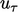, Eq. (7.10 ), we deﬁne friction temperature as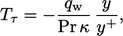(7.49)
The wall layer is then described by a dimensionless temperature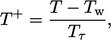(7.50)
where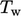is the ﬂuid temperature at the wall. Ignoring heat generation by viscous stresses, the proﬁle in the viscous sub-layer is described by the relation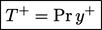(7.51)
The proﬁle in the inertial sub-layer is commonly described by a log law for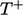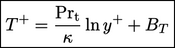(7.52)
The derivation of Eq. (7.51 ) and Eq. (7.52) assumes a constant heat ﬂux across the proﬁle, equating to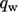at the wall. In the viscous sub-layer, the heat ﬂux is laminar so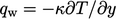and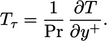(7.53)
This equation integrates between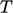at a distance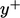from the wall to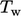at the wall, to yield Eq. (7.51). In the inertial layer, the heat ﬂux is assumed turbulent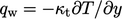and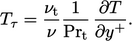(7.54)
Combining Eq. (6.24), Eq. (7.9) and Eq. (7.15 ) yields the ratio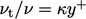. Substituting in Eq. (7.54 ) and integrating then leads to Eq. (7.52 ) where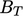is the constant of integration.

The constantis generally considered to be a function of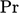. A reasonable approximation for this function is20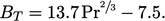(7.55)
Another function, commonly used in thermal wall functions, is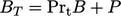, where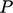is the function of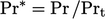:21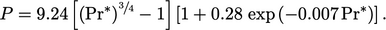(7.56)
The expression for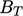uses the coeﬃcient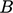from Eq. (7.11). These constants of integration are sometimes subsumed within the log function as a coeﬃcient “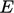” in the log law expressions, as the footnote on page 483 explains.
20Hermann Schlichting and Klaus Gersten, Boundary-layer theory, 2017.
21Chandra Jayatilleke, The inﬂuence of Prandtl number and surface roughness on the resistance of the laminar sub-layer to momentum and heat transfer, 1966.

Notes on CFD: General Principles - 7.13 Thermal boundary layers